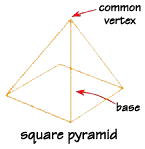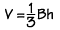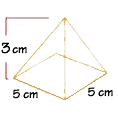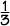Home    |    Teacher    |    Parents    |    Glossary    |    About UsThe pyramid is a famous shape primarily because of the ancient Egyptian pyramids. There are many mysteries surrounding these giant constructions, but one thing is certain--geometry was used to build them!

A pyramid is a polyhedron that has only one base. (The base is the "bottom" of the Egyptian pyramids.) The other faces are all congruent triangles, and they share a common vertex, which is the top point. The base can be any type of polygon. If the base is a triangle, then the pyramid has a total of four faces. The Egyptian pyramids have square bases and four triangles as faces.The volume of a pyramid is a measure of how much it would take to fill the shape. For a pyramid, the formula is:Where B is the area of the base figure, and h is the height from the base to the vertex. The volume is expressed in measurement units, cubed, like cubic inches. See if you can imagine little cubes filling up the interior space of the shape.

This formula is true for pyramids of any shape base. As long as you can find the area of the base and you know the height, you can calculate the volume.

What is the volume of a pyramid with a square base with sides of 5 cm, and a height of 3 cm?V =(5cm x 5cm) x 3 cm
Volume = 25 cm3

 Homework Help | Geometry | Three-dimensional figuresEmail this page to a friendSearch·  Space figures·  Prisms·  Pyramids·  Cylinders, cones     and spheresFirst Glance In Depth Examples WorkoutPyramids